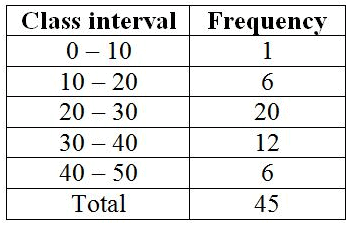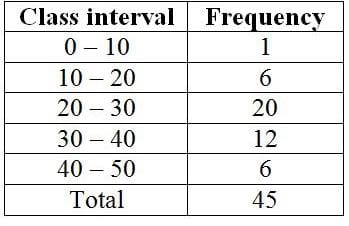# Test: Data Handling

## 10 Questions MCQ Test Advance Learner Course: Mathematics (Maths) Class 6 | Test: Data Handling

Description
Attempt Test: Data Handling | 10 questions in 10 minutes | Mock test for Class 7 preparation | Free important questions MCQ to study Advance Learner Course: Mathematics (Maths) Class 6 for Class 7 Exam | Download free PDF with solutions
QUESTION: 1

### The number of times an observation occurs in a data is called its

Solution:

Data available in an unorganised form are called raw data. The number of times a particular observation occurs in a given data is called its frequency.

QUESTION: 2

### In the class- interval 70-80, 80 is the

Solution:

Upper limit is the largest quantity in the interval. So 80 is the upper limit

QUESTION: 3

### The class mark of class 95- 100 is

Solution:

Class mark is the midpoint of the upper and lower limits.
so for the given problem; the class mark is
[(95+100)÷2] = 97.5
Thus class mark is 97.5

So option C is the correct answer.

QUESTION: 4

The difference between the upper and lower limit is called

Solution:

The difference between the upper and lower limit of a class interval is called the class size of the class interval.

QUESTION: 5

The mean weight of 100 students in a class is 46 kg. The mean weight of boys is 50 and of girls is 40 kg. Therefore, the number of boys is:

Solution:

Let number of boys are x and then number of girls = (100-x).
Thus,
50x+(100-x)*40 = 46*100
→ x = 60.
Number of boys = 60.

QUESTION: 6

Find the mean weight of the data set shown. 5 kg, 43 kg, 18 kg, 26 kg, 28 kg, 46 kg, 41 kg, 1 kg

Solution:

Mean =  Sum of mass weights / Total number of weighs

= (5 + 43 + 18 + 26 + 28 + 46 + 41 + 1)/8

= 26

QUESTION: 7

A kennel can accommodate 12 dogs that weigh (in pounds) 6, 14, 23, 17, 19, 27, 39, 7, 33, 4, 11, and 13. Find the range of their weights.

Solution:

The Range is the difference between the lowest and highest values.

Here the highest value is 39 and the lowest value is 4

So Range = Highest Value - Lowest Value

= 39 - 4 = 35

So option B is the correct answer.

QUESTION: 8

Find the mode for the data set, which shows the heights (in inches) of 10 students of Piyush’s class. 65, 60, 64, 61, 66, 67, 67, 67, 62, 77

Solution:

the mode of a set of numbers is the number that appears most often in the set.

Since the number 67 appears the most . So the mode of the given data is 67.

So option B is the correct answer.

QUESTION: 9

Following frequency distribution table shows marks (out of 50) obtained in English by 45 students of class VII. What is the size of class intervals?Solution:
QUESTION: 10

Which class has the highest frequency?Solution:Use Code STAYHOME200 and get INR 200 additional OFF Use Coupon Code